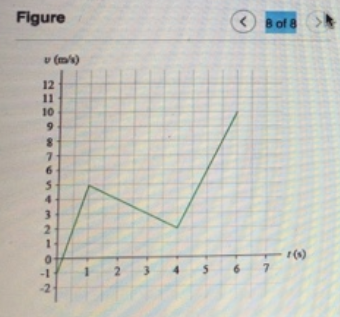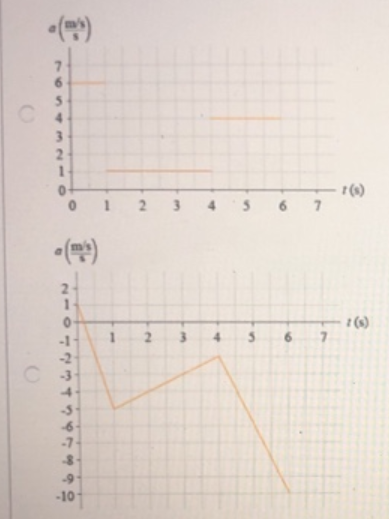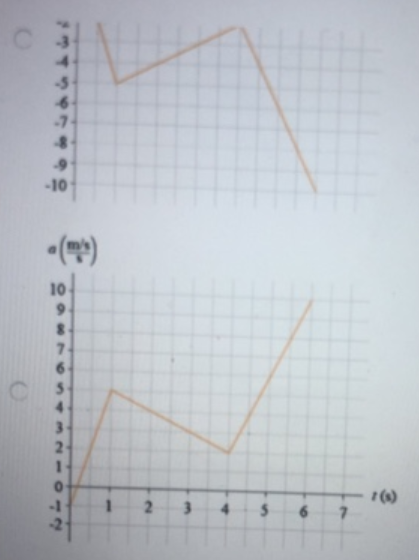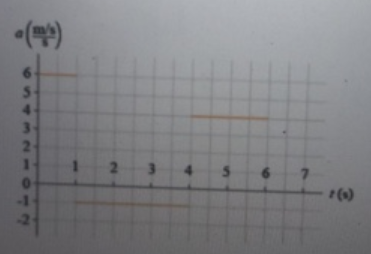# Problem: What is the correct acceleration vs. time graph for the velocity vs. time graph shown in Figure 8?

###### FREE Expert Solution

Acceleration is the slope of the velocity vs. time graph.

From 0 s to 1 s, the acceleration is:

a = [5 - (-1)]/(1 - 0) = 6 m/s2

95% (44 ratings)###### Problem DetailsWhat is the correct acceleration vs. time graph for the velocity vs. time graph shown in Figure 8?